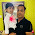## Wednesday, 5 August 2015

1.This solution is unique. It will be nice if you include the program in code tags :-)

2.I am a beginner learning data structures. Please let me know how did you derive to O(n2) complexity?

1.Hi,
If you see the program, There is a outer for loop "for(int i = 0; i < sudoku.length; i++)", which runs exactly length of sudoku(say n)in other work 9 times. Inside this for loop we have 3 internal for loop which runs exactly n/3 time.
so internal loop will be (n/3+n/3+n/3==n). So each value of out loop internal executes n time.
SO
1st iteration outer loop == n time internal loop

2nd iteration outer loop == n time internal loop

3rd iteration outer loop == n time internal loop
.
.
.
.
nth iteration outer loop == n time internal loop

Total ==> n+n+n+.........+n(n times)
n(1+1+1+..........+n times)
n(n)
O(n2)

3.